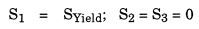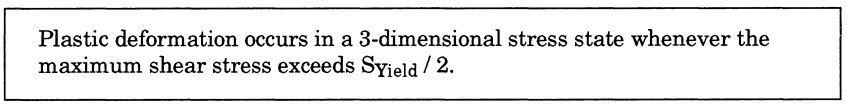top of page
Search

# 1.1.3 Failure Theories

To be useful, calculated stresses must be compared to material allowables. Material allowable stresses are related to strengths as determined by material uniaxial tensile tests, therefore calculated stresses must also be related to the uniaxial tensile test. This relationship can be developed by looking at available failure theories.There are three generally accepted failure theories which may be used to predict the onset of yielding in a material:

1. 1OCTAHEDRAL SHEAR, or VON MISES THEORY

2. MAXIMUM SHEAR, or TRESCA THEORY

3. MAXIMUM STRESS or RANKINE THEORY

These theories relate failure in an arbitrary three dimensional stress state in a material to failure in a the stress state found in a uniaxial tensile test specimen, since it is that test that is most commonly used to determine the allowable strength of commonly used materials. Failure of a uniaxial tensile test specimen is deemed to occur when plastic deformation occurs; i.e., when the specimen yields.

The three failure theories state: Octahedral Shear - Von Mises Theory:

"Failure occurs when the octahedral shear stress in a body is equal to the octahedral shear stress at yield in a uniaxial tension test."

The octahedral shear stress is calculated as:In a uniaxial tensile test specimen at the point of yield:Therefore the octahedral shear stress in a uniaxial tensile test specimen at failure is calculated as:Therefore, under the Von Mises theory:Maximum Shear Stress - Tresca Theory:

"Failure occurs when the maximum shear stress in a body is equal to the maximum shear stress at yield in a uniaxial tension test."

The maximum shear stress is calculated as:In a uniaxial tensile test specimen at the point of yield:In a uniaxial tensile test specimen at the point of yield:Therefore, under the Tresca theory:Maximum Stress - Rankine Theory

"Failure occurs when the maximum tensile stress in a body is equal to the maximum tensile stress at yield in a uniaxial tension test."

The maximum tensile stress is the largest, positive principal stress, Si. (By definition, S1 is always the largest of the principal stresses.)

In a uniaxial tensile test specimen at the point of yield:Therefore, under the Rankine theory:Introduction to Pipe Stress Analysis